# Conditional Transition Matrix

## Definition

A Conditional Transition Matrix (also Stressed Migration Matrix) is a Transition Matrix whose transition probabilities between different states are conditional on (given the information from) the realization of certain random variables (the Stress Scenario).

Irrespective of the manner by which it is derived, a conditional matrix is a modelled outcome (depends on Model Assumptions), in contrast with the Empirical Transition Matrix that reflects more or less directly the observed transition rates

## Model Construction

Model construction varies depending on the context. In Credit Risk Modelling context there are two broad categories

• Fitting Markov Chain models to market data that capture transition rates
• Fitting Markov Chain models to historical data (records) of state transitions

Models will typically invoke explanatory variables (covariates) which are linked to transition rates. We are then talking about "Dynamic Models of Transitions Rates"

Conditional migration probabilities can be derived for large collections of entities under the assumption of diversification of any idiosyncratic risk and the absence of unexplained variance. In such case specification of a set of common (systematic / macro) factors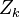$Z_k$ completely specifies the scenario. Conditional on systematic factor processes the random variables representing state transitions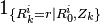${1}_{\{R^i_k=r | R^i_0 , Z_k\}}$

are independent and identically distributed. Their limiting sum converges to their expected value (law of large numbers)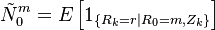$\tilde{N}^m_0 = {E} \left[ {1}_{\{R_k=r | R_0 = m, Z_k \}} \right]$

## Scenario Specification

Given a dynamic model of transition rates, conditioning a transition matrix involves:

• specifying the total time period (time horizon)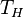$T_H$
• specifying the frequency (Temporal Grid)
• fixing the values of the model covariates (factors, drivers)$Z_k$ at the desired frequency and for the required duration

## Canonical (Gaussian) Model

This example is based on the canonical (Gaussian) model documented in Threshold Models. The transition thresholds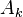$A_{k}$ for each period k are already known.

### Transition Drivers

Transitions of the i-th entity are assumed to be governed by a model of the type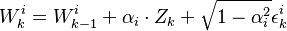$W_{k}^{i} = W_{k-1}^{i} + \alpha_i \cdot Z_k + \sqrt{1 - \alpha_i^2} \epsilon_{k}^{i}$

### Integration Method

The conditional rating transitions probabilities are obtained by numerical integration. All entities are assumed to be members of a homogeneous ensemble (we drop the index i). Let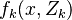$f_{k}(x, Z_k)$ denote the conditional probability density of the process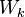$W_k$ that a given entity has not reached an absorbing state until period k-1.

### First Period

The first period density is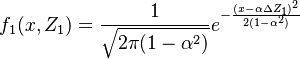$f_{1}(x, Z_1) = \frac{1}{\sqrt{2\pi (1-\alpha^2)}} e^{-\frac{(x - \alpha \Delta Z_1)^2}{2 (1 - \alpha^2)}}$

### Subsequent Periods

Then for every subsequent period the conditional density is obtained by convolution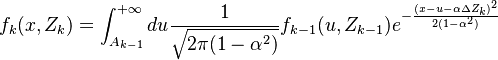$f_{k}(x, Z_k) = \int^{+\infty}_{A_{k-1}} du \frac{1}{\sqrt{2\pi (1 - \alpha^2)}} f_{k-1}(u, Z_{k-1}) e^{-\frac{(x- u - \alpha \Delta Z_k)^2}{2 (1 - \alpha^2)}}$

### Conditional Transition Probabilities

The cumulative conditional probability for any desired transition is then given by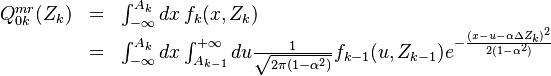$\begin{array}{lll} Q^{mr}_{0k}(Z_k) & = & \int^{A_{k}}_{-\infty} dx \, f_{k}(x, Z_k) \\ & = & \int^{A_{k}}_{-\infty} dx \int^{+\infty}_{A_{k-1}} du \frac{1}{\sqrt{2\pi (1 - \alpha^2)}} f_{k-1}(u,Z_{k-1}) e^{-\frac{(x- u - \alpha \Delta Z_k)^2}{2 (1 - \alpha^2)}} \end{array}$

## Properties

• A conditional transition matrix must satisfy the basic properties of a Transition Matrix
• When integrated over all possible scenarios the conditional transition matrix must reproduce the unconditional input. Symbolically, if F denotes the

(multivariate) distribution of the process Z: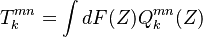$T^{mn}_{k} = \int dF(Z) Q^{mn}_{k}(Z)$